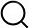# Also in the Article

Hierarchical network

Constructing graphs from genetic encodings
Sci Rep, Jun 24, 2021;

Procedure

Similar to the binary tree, a genetic rule-based heuristic for generating hierarchical networks relies on a motivated indexing of node identity14. The core module of a hierarchical network is a 5-clique, corresponding to n = 0, which requires at least b = 3 to encode. We label the node at the center ‘000’, and the four nodes on the ‘periphery’ to be ‘1XX’. In this way, the first bit of a 3-bit set corresponds to whether a node is in the center (0) or at the periphery (1). Thus, the n = 0 hierarchical network can be coded with the rules (1XX)O(1XX), which connects the peripheral nodes to each other, and $(1XX)O(0XX)$, which connects the periphery to the center.

The n = 1 hierarchical network is generated by making four copies of the 5-clique, and having the peripheral nodes of the copy connect to the center node of the original (Fig. S4b). This leads to a new definition of core and periphery: a node can be in the ‘core’, or original, clique, or in the peripheral, or copied, cliques, but also a node can be in the center or on the periphery within a clique. In this way, n = 1 can be coded easily with 6 bits, the first of which determine which clique a node is in, and the second of which determine where in the clique the node is present. For instance, 101000 is a central node in a peripheral clique, as the first three bits ‘101’ indicate its peripheral clique status, but ‘000’ as the second three bits corresponds to a central node. Alternatively, 000100 is a peripheral node in the central clique. To wire the system up properly, we introduce a separate clique wiring, as introduced in the previous paragraph, for every possible inner and outer clique in the system, such that we have rules of the type $(0001XX)O(0001XX)$, $(1001XX)O(1001XX)$, $(1011XX)O(1011XX)$, $(1101XX)O(1101XX)$, and $(1111XX)O(1111XX)$, as well as similar rules ending in ‘1XX’ on the source and ‘0XX’ on the destination. Then, each of the nodes that are ‘doubly’ peripheral connects back to the ‘doubly’ central node with rule $(1XX1XX)O(000000)$.

This process can be continued, such that we keep introducing Xs into the rules until Xs are present in the ‘outermost’ 3-bit set. This means that for a hierarchical network of depth n, we get $5n-1$ clique-forming rules, $5n-2$ rules that generate an n=1 hierarchy, and $5n-k-1$ rules that generate a n = k hierarchy. For instance, for an n = 2 hierarchical network (Fig. S4c), we had $52-1=5$ clique rules and $52-2=1$ n = 2 rules. This process is best illustrated in the supplementary code, which can create hierarchical networks of any depth, such as n = 3 (Fig. S4d), which was not present in the original paper on hierarchical networks14.

Q&A